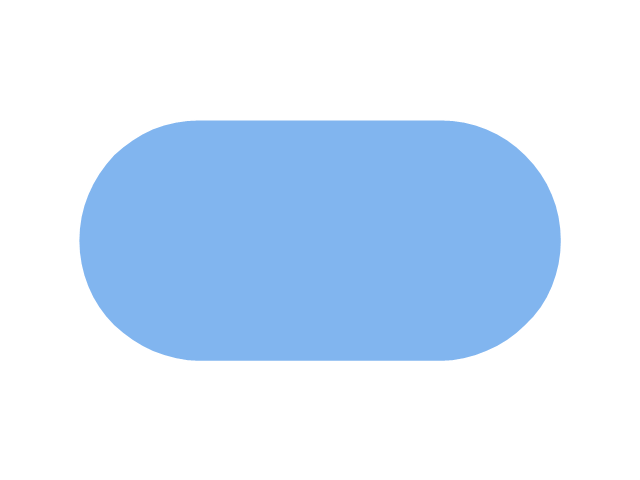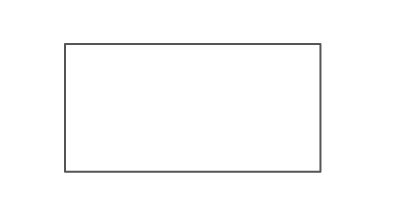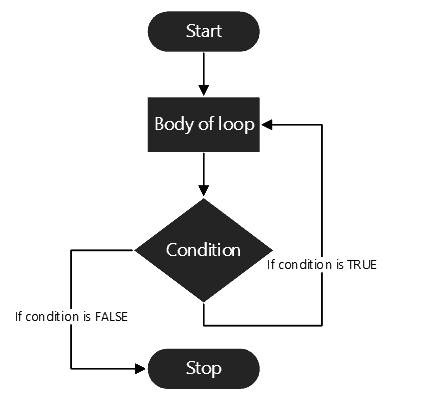Terms
Variables
Math
Flowchart Symbols
100

When I create a series of detailed, ordered steps that will always lead me (or anyone else) to the exact same result, I am creating an ______

Algorithm

100

Variables used to represent whole numbers are called _____

Integer

100

What is the correct way in computer science to multiply two numbers?
a) w= m X n
b) w= (m)(n)
c) w= m*n

c

100

What does this symbol represent?Start / End

200

This is the information that the user gives to the program.

Input

200

Can I do this?
a= "banana"
b=a

Yes.
200

What is the correct way in computer science to represent a square exponent?
a) y= x2
b) y= (x)(x)
c) y= x^2

c

200

What does this symbol represent?Input / Output

300

This is the information that the program sends/shows to the user.

Output

300

Can I do this?
a = "banana"
b= "yellow"
c= b+a

No

300

How can I get the 40% of my variable 'p'?
a) f = (p*40)/100
b) f= p - (p*0.6)
c) f= p*0.4
d) all of the above

d.

300

What does this symbol represent?Process

400

In a problem that needs my birth date to calculate my age, my birth date would be an  ____ variable.

Input

400

Find the mistake in the following statements:
C = a+b
a= c-b

Uppercase 'C'

400

The correct order of operations is:
a) Parentheses, Exponents, Multiplication, Division, Addition, Substraction
b) Parentheses, Multiplication, Exponents, Addition, Division, Substraction
c) Parentheses, Exponents, Addition, Multiplication, Division, Substraction

a.

400

What does this symbol represent?Decision

500

In a problem that needs my birth date to calculate my age, my age would be an  ____ variable.

Output

500

TRUE or FALSE:
*an incorrect explanation will not count!
When displaying plain text we put it inside quotation marks, however, text values in a variable, don't use quotation marks.

False

500

I want to calculate how much I would pay in a year with all of the following elements:
a monthly fee (m)
+ a 20% Christmas discount on December
+ a 25% discount on my birthday month.
+ a yearly subscription (y)

There is only one correct statement. Which one is it?
a) pay= (m*10)+(m*0.2)+(m-m*0.25)+y
b) pay = (m*12)+(m*0.8)+(m-m*0.25)+y
c) pay = (m*10)+(m*0.8)+(m-m*0.25)+y

c.

500

What does this structure represent?Cycle.

Click to zoom